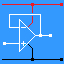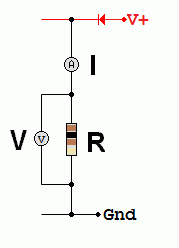RANDOM PAGE

SITE SEARCH

LOG
IN

HELP

# Power

This is the AQA version closing after June 2019. Visit the the version for Eduqas instead.

a

## Energy Measured in Joules, Power Measured in Watts

One Watt is the same as One Joule per Second.

Circuits consume energy from the power supply and produce energy at the output in the form of movement, light, sound, heat and so on. Circuits also produce waste heat energy.

b

## Power CalculationsIn the diagram, the power in Watts is dissipated as heat by the resistor.

To prevent overheating, the resistor power rating must be sufficient.

c

P = I V

P = V2 / R

P = I2 R

d

## Alternating Current Calculations

To calculate RMS power, use RMS values for the voltage and current.

Vrms = 0.7 Vpeak

Irms = 0.7 Ipeak

Prms = Irms Vrms

Prms = Vrms2 / R

Prms = Irms2 R

e

## Deriving Power Formulas

Ohm's law states that current, voltage and resistance are related in the following three ways ...

• R = V / I
• V = I R
• I = V / R

Starting with

• P = V I
• V = IR

and by substituting V you get ...

• P = I2 R

Starting with

• P = V I
• I = V / R

and by substituting I you get ...

• P = V2 / R

reviseOmatic V3     Contacts, ©, Cookies, Data Protection and Disclaimers Hosted at linode.com, London